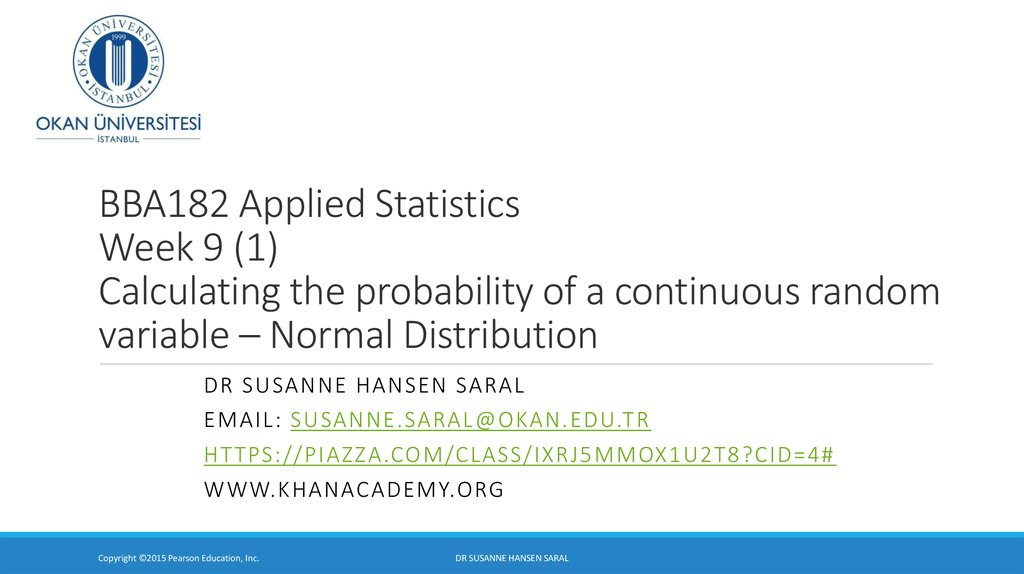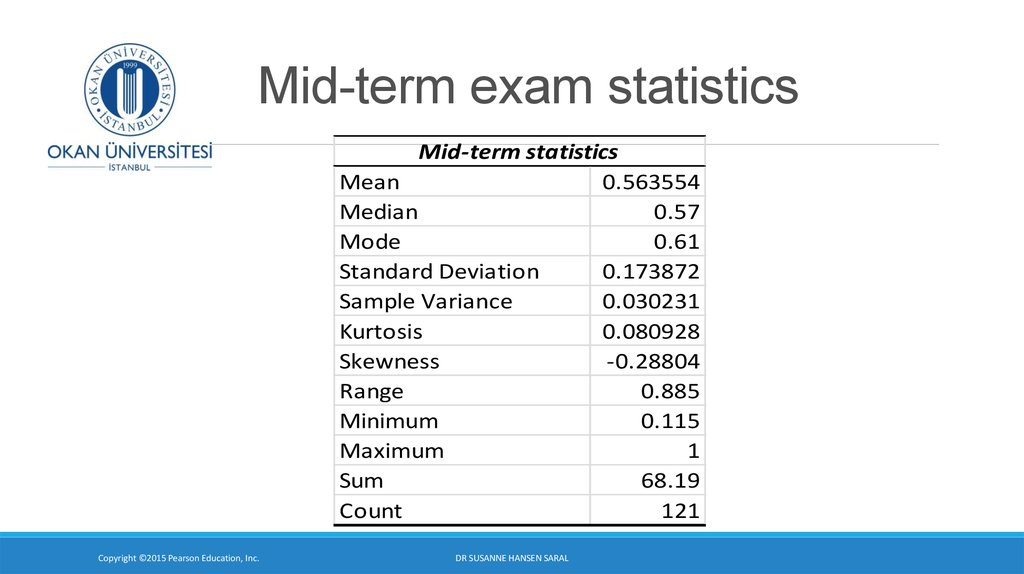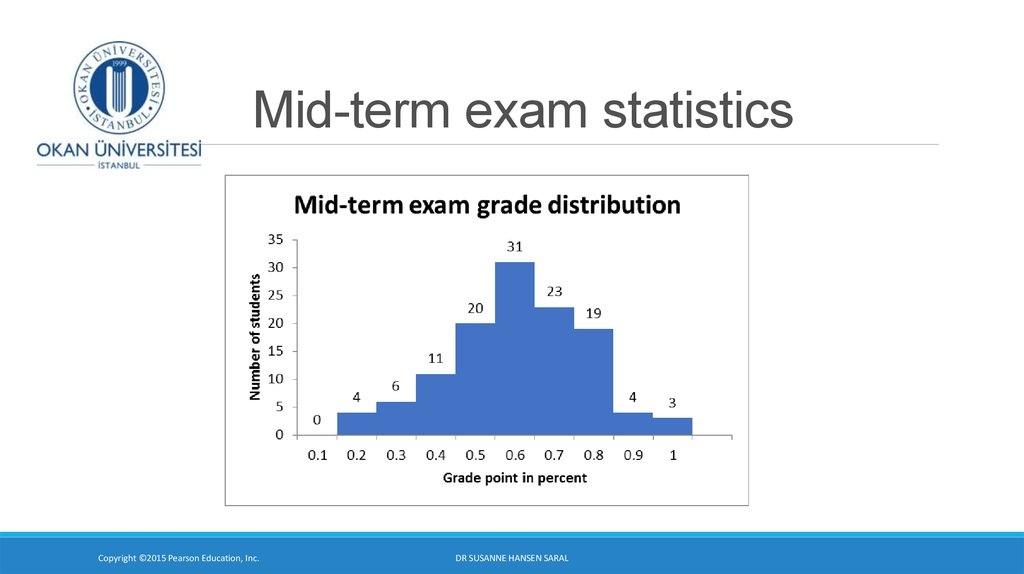# Calculating the probability of a continuous random variable – Normal Distribution. Week 9 (1)

## 1. BBA182 Applied Statistics Week 9 (1) Calculating the probability of a continuous random variable – Normal Distribution

DR SUSANNE HANSEN SARAL
EMAIL: [email protected]
HT TPS://PIAZZA.COM/CLASS/IXRJ5MMOX1U2T8?CID=4#
DR SUSANNE HANSEN SARAL

## 2. Mid-term exam statistics

Mid-term statistics
Mean
0.563554
Median
0.57
Mode
0.61
Standard Deviation
0.173872
Sample Variance
0.030231
Kurtosis
0.080928
Skewness
-0.28804
Range
0.885
Minimum
0.115
Maximum
1
Sum
68.19
Count
121
DR SUSANNE HANSEN SARAL

## 3. Mid-term exam statistics

DR SUSANNE HANSEN SARAL

## 4. Continuous random variable

A continuous random variable can assume any value in an interval
on the real line or in a collection of intervals.
It is not possible to talk about the probability of the random variable
assuming a particular value, because the probability will be close to
0.
assuming a value within a given interval.
DR SUSANNE HANSEN SARAL

## 5. Calculating probabilities of continuous random variables

F(b) P(X b)
a
μ
b
x
a
μ
b
x
a
μ
b
x
F(a) P(X a)
P(a X b) F(b) F(a)
HALL
Ch. 5-5

## 6. The Standard Normal Distribution – z-values

Any normal distribution, F(x) (with any mean and
standard deviation combination) can be transformed
into the standardized normal distribution F(z), with
mean 0 and standard deviation 1
f(Z)
Z ~ N(0 ,1)
1
0
Z
We say that Z follows the standard normal distribution.
HALL
Ch. 5-6

## 7. Procedure for calculating the probability of x using the Standard Normal Table

For m = 100, s = 15, find the probability that X is less than 130 = P(x < 130)
Transforming x - random variable into a z - standard random variable:
Z=
X -m
s
130-100
=
15
m = 100
s = 15
30
=
= 2 std dev
15
P(X < 130)
|
55
FIGURE 2.9
– Normal Distribution
|
70
|
85
|
100
|
115
|
130
|
145
|
1
|
2
|
3
X = IQ
m
|
–3
|
–2
|
–1
DR SUSANNE HANSEN SARAL
|
0
Z=
X -m
s

## 8. Procedure for calculating the probability of x using the Standard Normal Table (continued)

Step 2
• Look up the probability from the table of normal curve areas
• Column on the left is Z value
• Row at the top has second decimal places for Z values
DR SUSANNE HANSEN SARAL

## 9. Using the Standard Normal Table

TABLE 2.10 – Standardized Normal Distribution (partial)
Z
1.8
1.9
2.0
2.1
2.2
AREA UNDER THE NORMAL CURVE
0.00
0.01
0.02
0.03
0.96407
0.96485
0.96562
0.96638
0.97128
0.97193
0.97257
0.97320
0.97725
0.97784
0.97831
0.97882
0.98214
0.98257
0.98300
0.98341
0.98610
0.98645
0.98679
0.98713
For Z = 2.00
P(X < 130)
P(X > 130)
= P(Z < 2.00) = 0.97725
= 1 – P(X ≤ 130) = 1 – P(Z ≤ 2)
= 1 – 0.97725 = 0.02275
DR SUSANNE HANSEN SARAL

## 10. P(z < + 2) = P(z > -2) = .9772

P(z < + 2) = P(z > -2) = .9772
In probability terms, a z-score of -2.0 and +2.0 has the same probability,
because they are mirror images of each other.
If we look for the z-score 2.0 in the table we find a value of 9772.
DR SUSANNE HANSEN SARAL

## 11.

The Standard Normal Table
To find the probability of: P (z > 1) and P (z < -1) we will use the
complement rule:
P( z > 1.0) = 1 - .8413 = 0.1587
.8413
.1587
0
Z
1.00
.8413
P( z < - 1.0) = 1 - .8413 = 0.1587
.1587
-1.00
DR SUSANNE HANSEN SARAL
0
Z

## 12. Finding the probability of z-scores with two decimals and graph the probability

P ( z < + 0.55) = 0.7088
or 70.88 %
P (z > + .55) = 1.0 – 0.7088 = 0.2912 or 29.12%
P ( z > - 0.55) = 0.7088 or 70.88 %
P ( z < - 0.55) = 1.0 - .7088 = 0.2912 or 29.12 %
P ( z < + 1.65) = 0.9505 or 95.05 %
P (z > + 1.65) = 1.0 – 0.9505 = 0.0495 or 4.96 %
P( z > - 2.36) = .9909 or 99.09 %
P ( z < + 2.36) = .9909 or 99.09 %